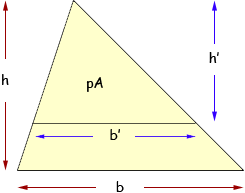Dear Math Central, I'm a creative type who needs to find how you can determine the horizontal, relative percentages within a triangle. Attached is a pdf showing approximately the 50% line, but I'd like to know how to determine any percentage (horizontally) within a triangle . I know that if I put a horizontal line though the height of the triangle I won't be showing 50% above and 50% below. The bottom part has to be larger (correct me if I'm wrong). I'm not concerned about height as I am wanting to break down the percentages into 60% at the bottom, 25% in the middle and 15% at the top. Thanks in advance for your help. Sincerely, Ken Hi Ken, I produced a diagram much like the one you sent and then I put some labels on it.The initial triangle has base of length b and height h so the area is given by A = 1/2bh The horizontal line cuts off a certain proportion of the area above the line. I want to call this proportion p. (I would rather work with proportions than percentages.) The horizontal cut-off line is of length b' and the triangle above this line has height h'. Thus the area of the triangle above the cut-off line is given by pA = 1/2b'h' Substitute A from the equation above to get p1/2bh = 1/2b'h' Thus pbh = b'h' or p = b'/bh'/h   The original triangle and the triangle above the cut-off line are similar so  b'/b =  h'/h  and hence   p = h'/hh'/h  = (h'/h)2 Finally h' = √ph Thus in your example you want 15% of the area above the cut-off line so p = 0.15 and √0.15 = 0.39. Thus draw the cut-off line so that the height of the triangle above the cut-off line is 39% of the height of the original triangle. The next cut-off line is to leave 40% of the area of the original triangle above it so here p = 0.40 and √0.40 = 0.63. Thus draw the next cut-off line so that the height of the triangle above this cut-off line is 63% of the height of the original triangle. Penny